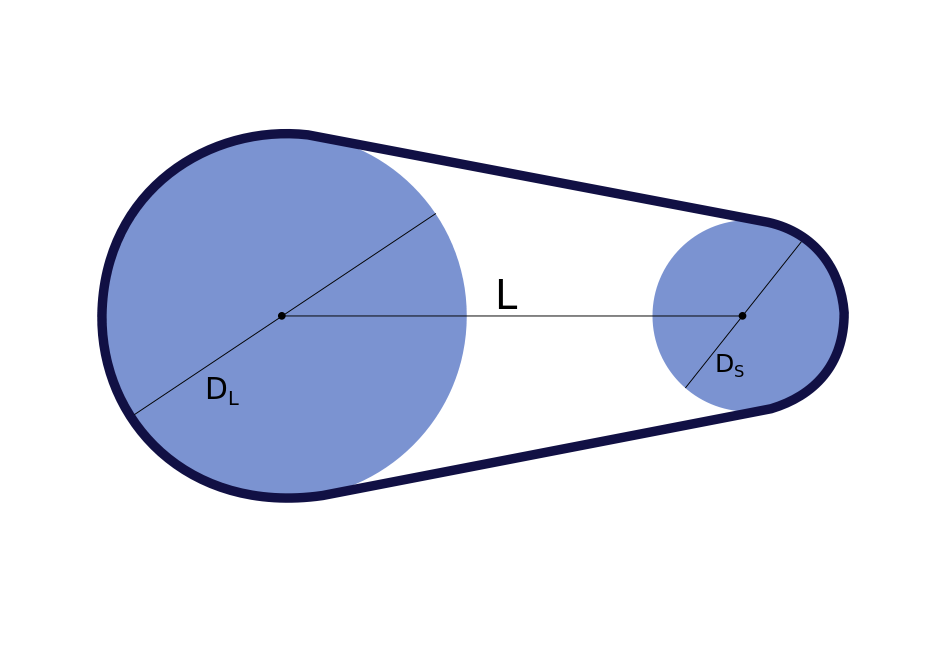# Belt Length Calculator

Created by Julia Żuławińska
Reviewed by Bogna Szyk and Jack Bowater
Last updated: Jan 25, 2023

Welcome to the belt length calculator! If you need to determine the length of any belt - be it a timing, ribbed, flat, or v-belt - you are in the right place. In the following text, we will introduce you to the belt length formula and show you how to use it.

Check out this pulley calculator to find out more about pulley RPM, speed, and belt tension.

## Two pulley system - belt length

Pulleys can trace their origins to before 200 B.C. Originally, they were used to lift buckets of water from wells, rivers, etc. Nowadays they are everywhere - in clocks mechanisms, on construction sites, and in elevators. And that's just the tip of the iceberg. System like this are used to transmit torque (or power) across axles, providing mechanical advance.Let's learn how to calculate the length of a belt in a two-pulley system with differing diameters of pulleys. You can do it by inputting the distance between the axles of the pulleys and their diameters into the following formula:

$\footnotesize \begin{split} &\text{Belt length} = \\ &\quad ((\text{D}_\text{L} + \text{D}_\text{S}) \cdot \frac{π}{2}) \\ &\quad +(\text{D}_\text{L} - \text{D}_\text{S}) \cdot arcsin(\frac{\text{D}_\text{L} - \text{D}_\text{S}} {2\text{L}}) \\ &\quad+ 2 \sqrt{(\text{L}^2− 0.25 \cdot (\text{D}_\text{L} - \text{D}_\text{S})^2)} \end{split}$

, where:

• $\small \text{D}_\text{L}$ is the diameter of the large pulley;
• $\small \text{D}_\text{S}$ is the diameter of the smaller pulley; and
• $\small \text{L}$ is the distance between the pulley axles.

With our belt length calculator, you can also calculate the distance between the centers of two pulleys for a given belt length.

## How to compute like our belt length calculator?

1. Let's say that you own a factory and you need to calculate a v-belt length. The diameters of the pulleys are 15 and 30 cm, and you want the distance between them to be 1.5 meters.
• $\small \text{D}_\text{L} = 30\space cm = 0.3\space m$
• $\small \text{D}_\text{S} = 15\space cm = 0.15\space m$
• $\small \text{L} = 1.5 \space m$
1. Input the values into the equation. Note, that the units are not relevant as long as they're the same for all variables.
$\footnotesize \begin{split} &\text{Belt length} = \\ &\quad ((0.3 + 0.15) \cdot \frac{π}{2}) \\ &\quad +(0.3 - 0.15) \cdot arcsin(\frac{0.3 - 0.15} {2\cdot 1.5}) \\ &\quad+ 2 \sqrt{(1.5^2− 0.25 \cdot (0.3 - 0.15)^2)} \\ &\quad= \bold{3.71}\space \bold m \end{split}$

Simple, right? But, did you know that there is an even easier solution to your belt length problem? And it doesn't have trigonometric functions or square roots!

## Another belt length formula?

There is a different belt length formula common among engineers. It's an approximation of the one given above:

$\footnotesize \begin{split} &\text{Belt length} = \\ &\frac{π}{2}(\text{D}_\text{L} + \text{D}_\text{S}) + 2\text{L} +\frac{(\text{D}_\text{L} - \text{D}_\text{S})^2}{4\text{L}} \\ \end{split}$

, where:

• $\small \text{D}_\text{L}$ is the diameter of the large pulley;
• $\small \text{D}_\text{S}$ is the diameter of the smaller pulley; and
• $\small \text{L}$ is the distance between the pulley axles.

You can find the belt length calculated with this equation by clicking on the Advanced mode button.

It works well for two pulleys of a similar size or ones that are further apart. The estimation fails when one pulley is much larger than the other and they are close together. However, such cases are uncommon in engineering, as they can lack the adequate drive. As engineers didn't always have a belt length calculator at hand, they used a simplified equation to calculate faster.

Interested in mechanics? The mechanical advantage calculator will ease you into the world of simple machines!

## FAQ

### How to calculate a conveyor belt lenght?

To calculate a conveyor belt length:

1. Check the diameters of the two pulleys you have.
2. Measure the distance between the pulley axles.
3. Use our belt length calculator to find the belt length in seconds.

### Does belt length affect speed?

While the length of a belt doesn't affect speed, its thickness does. That's why it's crucial to choose the right belt type for the pulleys you're using.

### What types of belts are there?

Belts can have different shapes and can be made from different materials. The type of belt you choose depends on the application. You can get the following:

• Flat belt;
• V belt;
• Circular belt;
• Timing belt;
• Spring belt;
• Ribbed belt;
• Film belt;
• And more.
Julia ŻuławińskaDiameter of the large pulley
in
Diameter of the small pulley
in
Pulley centre distance
in
Belt length
in
People also viewed…

### Density to mass

This density to mass calculator uses the density and volume of an object to calculate its mass.

### Helium balloons

Wondering how many helium balloons it would take to lift you up in the air? Try this helium balloons calculator! 🎈

### Steps to calories

Steps to calories calculator helps you to estimate the total amount to calories burned while walking.

### Thrust to weight ratio

Estimate the thrust to weight ratio for real aircraft or your designs using the thrust to weight ratio calculator.